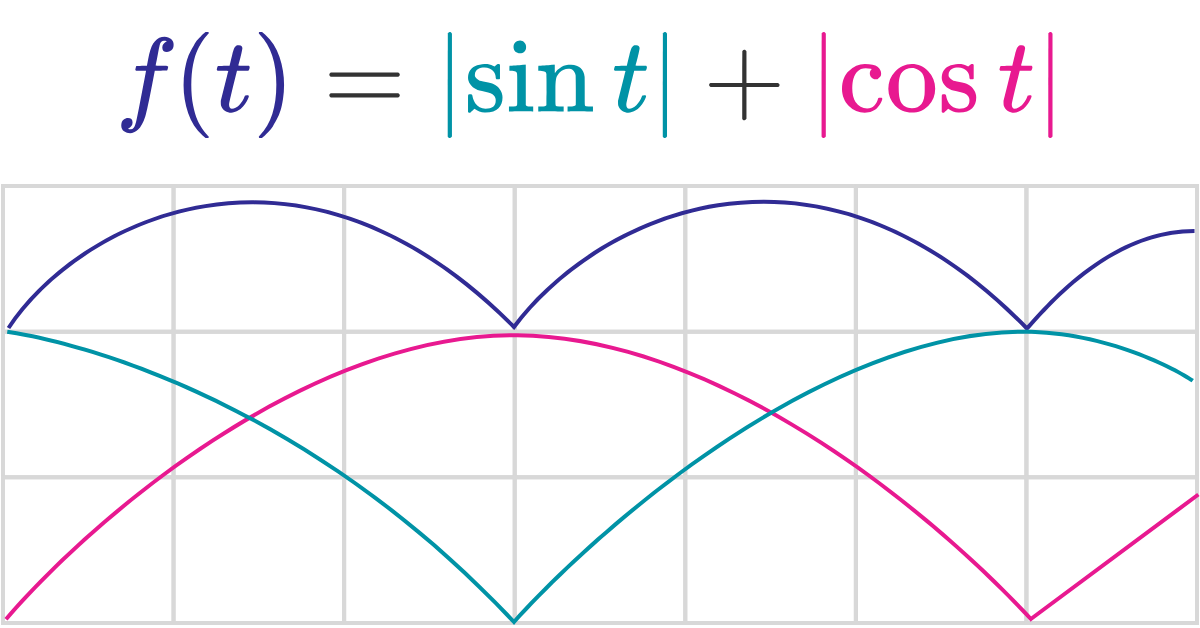# You call it Time period I call it a Question

Find the fundamental period of $f(t)=\left|\sin t\right|+\left|\cos t\right|.$Note: A function has a period of $T$ if $f(t) = f(t+T)$ for all $t$. The fundamental period is the smallest positive period.

×